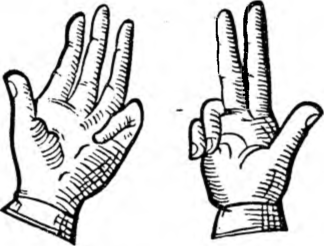# Multiplication on the fingers

Multiplication on the fingers

In discussing the wonders of the nine, we have provided an excellent way of finger-multiplication by this number.

A 17th-century Syrian author, named Beha-Eddin (1547-1622), in his book, very popular in Persia and India, under the title Khelasat as hissab (About the essence of the accounts) gives a slightly different one, but also an ingenious way of finger-multiplication by other numbers, essential for those, what they do not want or cannot learn the multiplication tables above 5.

Who got the secret, how much is 2 • 2, 2 • 3 and so on up to 5 • 5, he does not need to go higher in this difficult science, because his fingers are enough for more complicated multiplications.

Suppose, that you need to do multiplication 9 • 8.

But 9 = 5 + 4, a 8 = 5 + 3, it means
9 • 8 = (5 + 4) • (5 + 3).It is therefore necessary to raise 4 fingers on one hand and 3 fingers on the other hand. Sum of fingers raised (4 + 3) will indicate the number of tens of the product (7), and the unity of the product is achieved by multiplying the number of bent fingers of one hand by the number of such fingers of the other hand: 1 • 2 = 2.

So finally 9 • 8 = 72.

When multiplying 8 • 7, what gives (5 + 3) • (5 + 2), should be picked up with one hand 3 inches, and on the other 2 and bend the other fingers. The sum of outstretched fingers 3 + 2 = 5 it will be the number of tens, and the product of bent fingers 2 • 3 = 6 it will be the number of units of the result sought. Together will be 56. This is the difficult case of multiplication.

And yet, .. . however, it is probably better to just learn the multiplication tables.